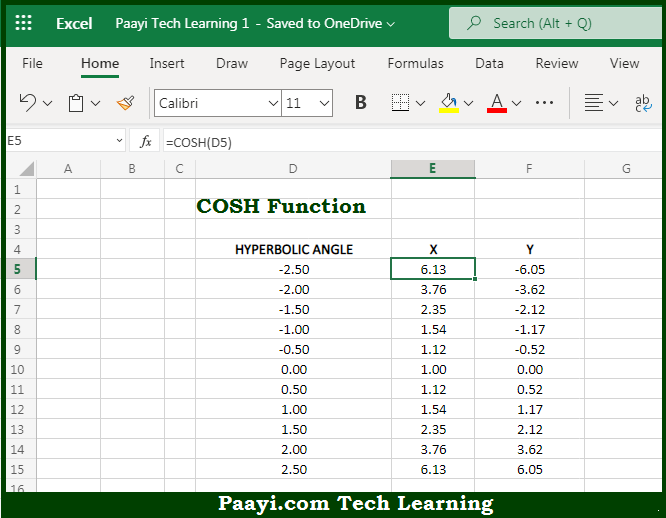# Learn How to Use Microsoft Excel COSH Function

Written by | 0 Comments | 647 Views

In this article, you will learn how to use the Microsoft Excel COSH function and its prime function in Microsoft Excel. You will also get to know the Microsoft Excel COSH function return value and syntax with the help of some examples.

Microsoft Excel COSH Function

The main purpose of the Microsoft Excel COSH function is to get the hyperbolic cosine of the number. That implies, with the help of the COSH function you can able to return the hyperbolic cosine of the given number. In geometry, the hyperbolic angle is defined as a ray from the origin of the coordinate system that passes through a point on the hyperbola. The area formed by the hyperbolic angle is equal to one-half the hyperbolic angle. So, with the help of the COSH function, you can able to get the hyperbolic cosine of the number.

Return Value of COSH Function

The return value will be the x-component of the hyperbolic angle.

Syntax of COSH Function

=COSH(number)

Where the arguments:

• number: This is the hyperbolic angle.

## How to Use Microsoft Excel COSH Function?So we know that Microsoft Excel COSH function you can able to get the hyperbolic cosine of the number. That implies, with the help of the COSH function you can able to return the hyperbolic cosine of the given number. In geometry, the hyperbolic angle is defined as a ray from the origin of the coordinate system that passes through a point on the hyperbola. The area formed by the hyperbolic angle is equal to one-half the hyperbolic angle. So, with the help of the COSH function, you can able to get the hyperbolic cosine of the number.